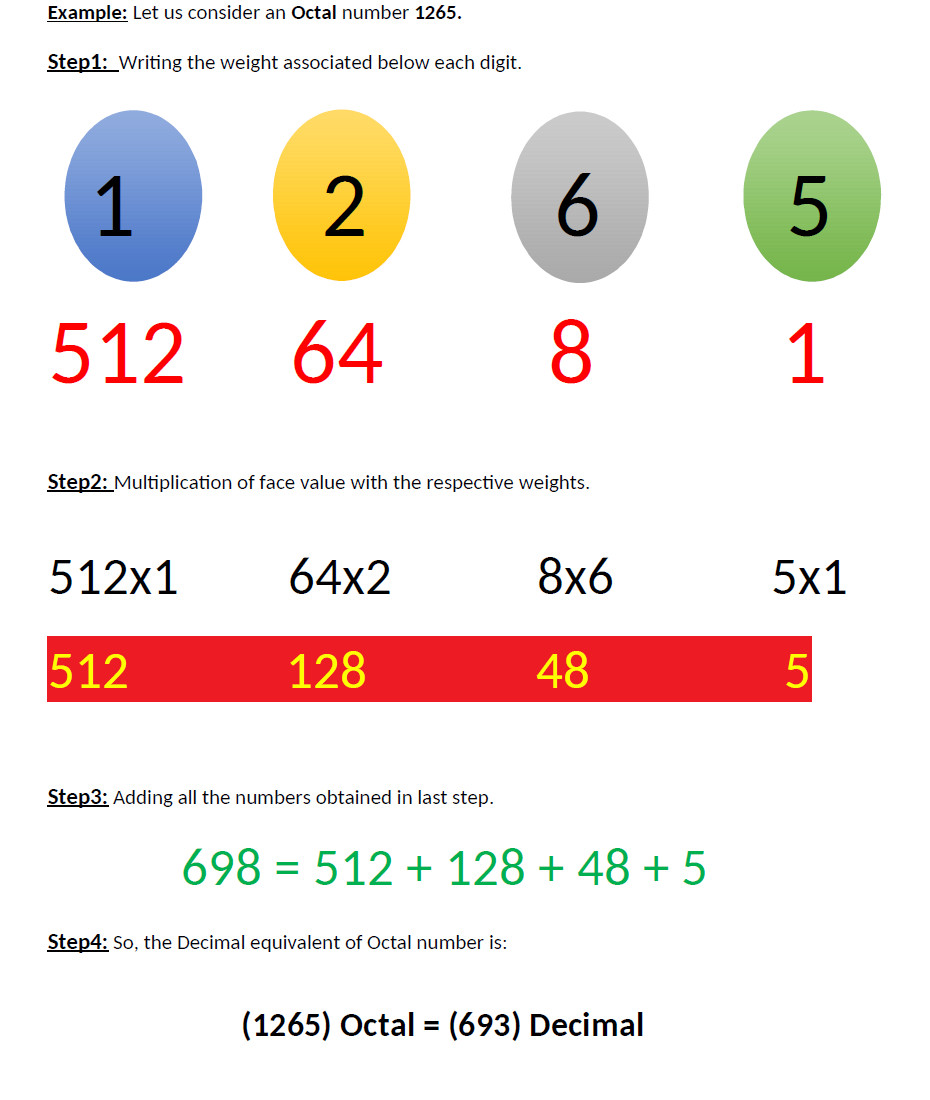Enter Decimal OR Octal Number:
Result

## 十进制到八进制数字系统的转换。

• 第1步：用小数除以8，记下余数并给它赋值R1。同样地，注意商，并给它分配值Q1。
• 第二步：现在用Q1除以8，记下余数和商。将R2和Q2的值分配给本步骤中得到的余数和商。
• 第三步：重复这个序列，直到你得到商的值（Qn）等于0。
• 第四步：八进制数字将看起来像这样: Rn R(n-1) R(n-2) ……………………... R3 R2 R1

1. 2181 / 8 = ( 272 x 8 ) + 5 ………………………………………... R1 = 5 Q1 = 272
2. 272 / 8 = ( 34 x 8 ) + 0 ……………………………………….. R2 = 0 Q2 = 34
3. 34 / 8 = ( 4 x 8 ) + 2 ………………………………………... R3 = 2 Q3 = 4
4. 4 / 8 = ( 0 x 8 ) + 4 ………………………………………... R4 = 4 Q4 = 0

(2181) Decimal = (4205) Octal

## 八进制转换为二进制数字系统。

• 第1步：在八进制数字的每个数字下面写下8的重量。
• 第二步：现在将每个数字与该位置或数字索引的相关权重相乘。
• 第三步：将上一步乘法后得到的所有数字相加。
• 第四步：最后一步得到的数字是八进制数字的十进制等值。